# Clustering Algorithm by Vishal.pdf

21 de Feb de 2023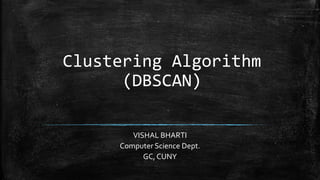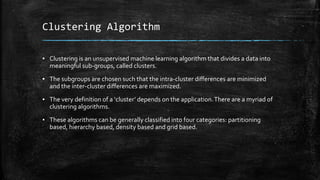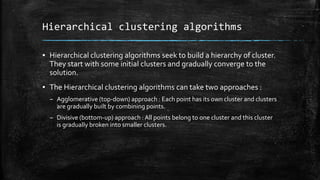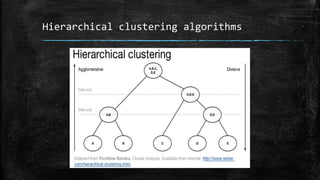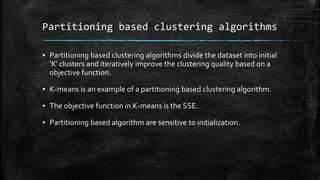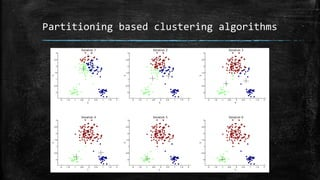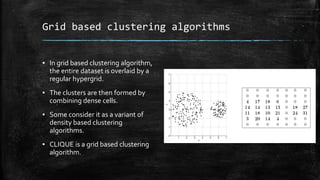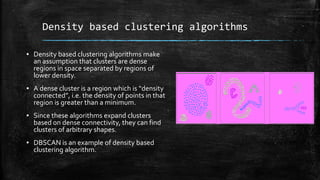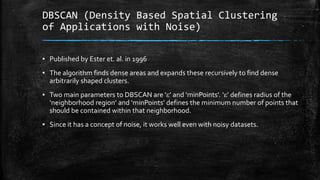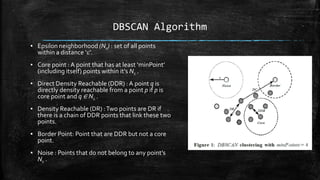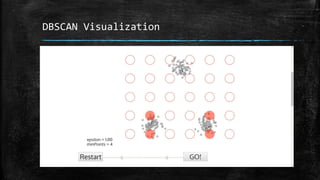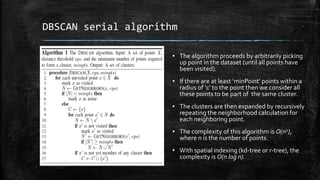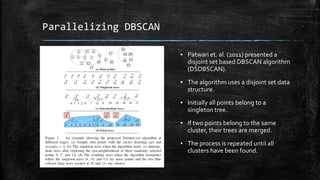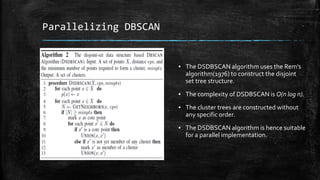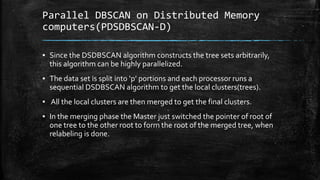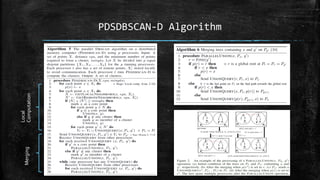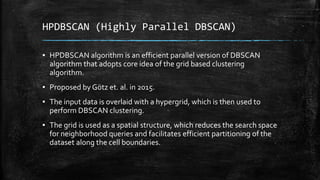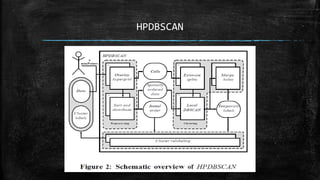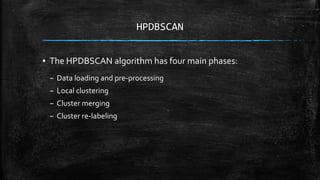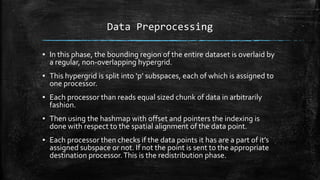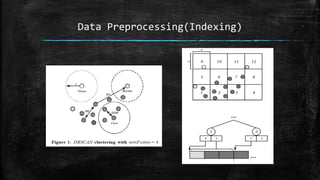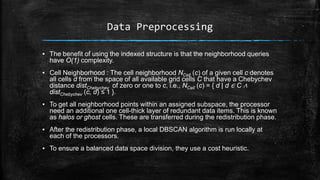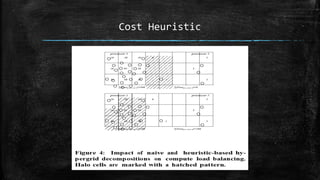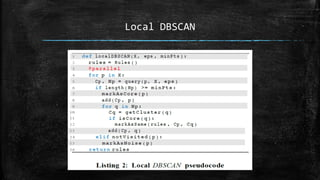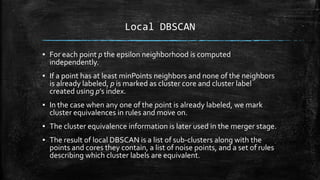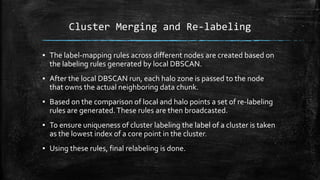1 de 27

### Clustering Algorithm by Vishal.pdf

• 2. Clustering Algorithm ▪ Clustering is an unsupervised machine learning algorithm that divides a data into meaningful sub-groups, called clusters. ▪ The subgroups are chosen such that the intra-cluster differences are minimized and the inter-cluster differences are maximized. ▪ The very definition of a ‘cluster’ depends on the application.There are a myriad of clustering algorithms. ▪ These algorithms can be generally classified into four categories: partitioning based, hierarchy based, density based and grid based.
• 3. Hierarchical clustering algorithms ▪ Hierarchical clustering algorithms seek to build a hierarchy of cluster. They start with some initial clusters and gradually converge to the solution. ▪ The Hierarchical clustering algorithms can take two approaches : – Agglomerative (top-down) approach : Each point has its own cluster and clusters are gradually built by combining points. – Divisive (bottom-up) approach : All points belong to one cluster and this cluster is gradually broken into smaller clusters.
• 5. Partitioning based clustering algorithms ▪ Partitioning based clustering algorithms divide the dataset into initial ‘K’ clusters and iteratively improve the clustering quality based on a objective function. ▪ K-means is an example of a partitioning based clustering algorithm. ▪ The objective function in K-means is the SSE. ▪ Partitioning based algorithm are sensitive to initialization.
• 7. Grid based clustering algorithms ▪ In grid based clustering algorithm, the entire dataset is overlaid by a regular hypergrid. ▪ The clusters are then formed by combining dense cells. ▪ Some consider it as a variant of density based clustering algorithms. ▪ CLIQUE is a grid based clustering algorithm.
• 8. Density based clustering algorithms ▪ Density based clustering algorithms make an assumption that clusters are dense regions in space separated by regions of lower density. ▪ A dense cluster is a region which is “density connected”, i.e. the density of points in that region is greater than a minimum. ▪ Since these algorithms expand clusters based on dense connectivity, they can find clusters of arbitrary shapes. ▪ DBSCAN is an example of density based clustering algorithm.
• 9. DBSCAN (Density Based Spatial Clustering of Applications with Noise) ▪ Published by Ester et. al. in 1996 ▪ The algorithm finds dense areas and expands these recursively to find dense arbitrarily shaped clusters. ▪ Two main parameters to DBSCAN are ‘ε’ and ‘minPoints’. ‘ε’ defines radius of the ‘neighborhood region’ and ‘minPoints’ defines the minimum number of points that should be contained within that neighborhood. ▪ Since it has a concept of noise, it works well even with noisy datasets.
• 10. DBSCAN Algorithm ▪ Epsilon neighborhood (Nε) : set of all points within a distance ‘ε’. ▪ Core point : A point that has at least ‘minPoint’ (including itself) points within it’s Nε . ▪ Direct Density Reachable (DDR) : A point q is directly density reachable from a point p if p is core point and q ∈ Nε . ▪ Density Reachable (DR) :Two points are DR if there is a chain of DDR points that link these two points. ▪ Border Point: Point that are DDR but not a core point. ▪ Noise : Points that do not belong to any point’s Nε .
• 12. DBSCAN serial algorithm ▪ The algorithm proceeds by arbitrarily picking up point in the dataset (until all points have been visited). ▪ If there are at least ‘minPoint’ points within a radius of ‘ε’ to the point then we consider all these points to be part of the same cluster. ▪ The clusters are then expanded by recursively repeating the neighborhood calculation for each neighboring point. ▪ The complexity of this algorithm is O(n2), where n is the number of points. ▪ With spatial indexing (kd-tree or r-tree), the complexity is O(n log n).
• 13. Parallelizing DBSCAN ▪ Patwari et. al. (2011) presented a disjoint set based DBSCAN algorithm (DSDBSCAN). ▪ The algorithm uses a disjoint set data structure. ▪ Initially all points belong to a singleton tree. ▪ If two points belong to the same cluster, their trees are merged. ▪ The process is repeated until all clusters have been found.
• 14. Parallelizing DBSCAN ▪ The DSDBSCAN algorithm uses the Rem’s algorithm(1976) to construct the disjoint set tree structure. ▪ The complexity of DSDBSCAN is O(n log n). ▪ The cluster trees are constructed without any specific order. ▪ The DSDBSCAN algorithm is hence suitable for a parallel implementation.
• 15. Parallel DBSCAN on Distributed Memory computers(PDSDBSCAN-D) ▪ Since the DSDBSCAN algorithm constructs the tree sets arbitrarily, this algorithm can be highly parallelized. ▪ The data set is split into ‘p’ portions and each processor runs a sequential DSDBSCAN algorithm to get the local clusters(trees). ▪ All the local clusters are then merged to get the final clusters. ▪ In the merging phase the Master just switched the pointer of root of one tree to the other root to form the root of the merged tree, when relabeling is done.
• 17. HPDBSCAN (Highly Parallel DBSCAN) ▪ HPDBSCAN algorithm is an efficient parallel version of DBSCAN algorithm that adopts core idea of the grid based clustering algorithm. ▪ Proposed by Götz et. al. in 2015. ▪ The input data is overlaid with a hypergrid, which is then used to perform DBSCAN clustering. ▪ The grid is used as a spatial structure, which reduces the search space for neighborhood queries and facilitates efficient partitioning of the dataset along the cell boundaries.
• 19. HPDBSCAN ▪ The HPDBSCAN algorithm has four main phases: – Data loading and pre-processing – Local clustering – Cluster merging – Cluster re-labeling
• 20. Data Preprocessing ▪ In this phase, the bounding region of the entire dataset is overlaid by a regular, non-overlapping hypergrid. ▪ This hypergrid is split into ‘p’ subspaces, each of which is assigned to one processor. ▪ Each processor than reads equal sized chunk of data in arbitrarily fashion. ▪ Then using the hashmap with offset and pointers the indexing is done with respect to the spatial alignment of the data point. ▪ Each processor then checks if the data points it has are a part of it’s assigned subspace or not. If not the point is sent to the appropriate destination processor.This is the redistribution phase.
• 22. Data Preprocessing ▪ The benefit of using the indexed structure is that the neighborhood queries have O(1) complexity. ▪ Cell Neighborhood : The cell neighborhood NCell (c) of a given cell c denotes all cells d from the space of all available grid cells C that have a Chebychev distance distChebychev of zero or one to c, i.e., NCell (c) = { d | d ∈ C ∧ distChebychev (c, d) ≤ 1 }. ▪ To get all neighborhood points within an assigned subspace, the processor need an additional one cell-thick layer of redundant data items. This is known as halos or ghost cells. These are transferred during the redistribution phase. ▪ After the redistribution phase, a local DBSCAN algorithm is run locally at each of the processors. ▪ To ensure a balanced data space division, they use a cost heuristic.
• 25. Local DBSCAN ▪ For each point p the epsilon neighborhood is computed independently. ▪ If a point has at least minPoints neighbors and none of the neighbors is already labeled, p is marked as cluster core and cluster label created using p’s index. ▪ In the case when any one of the point is already labeled, we mark cluster equivalences in rules and move on. ▪ The cluster equivalence information is later used in the merger stage. ▪ The result of local DBSCAN is a list of sub-clusters along with the points and cores they contain, a list of noise points, and a set of rules describing which cluster labels are equivalent.
• 26. Cluster Merging and Re-labeling ▪ The label-mapping rules across different nodes are created based on the labeling rules generated by local DBSCAN. ▪ After the local DBSCAN run, each halo zone is passed to the node that owns the actual neighboring data chunk. ▪ Based on the comparison of local and halo points a set of re-labeling rules are generated.These rules are then broadcasted. ▪ To ensure uniqueness of cluster labeling the label of a cluster is taken as the lowest index of a core point in the cluster. ▪ Using these rules, final relabeling is done.